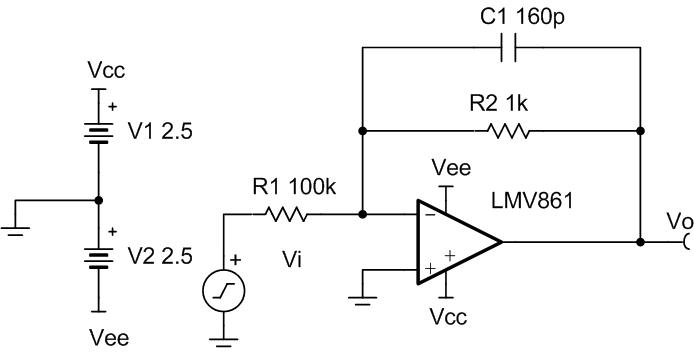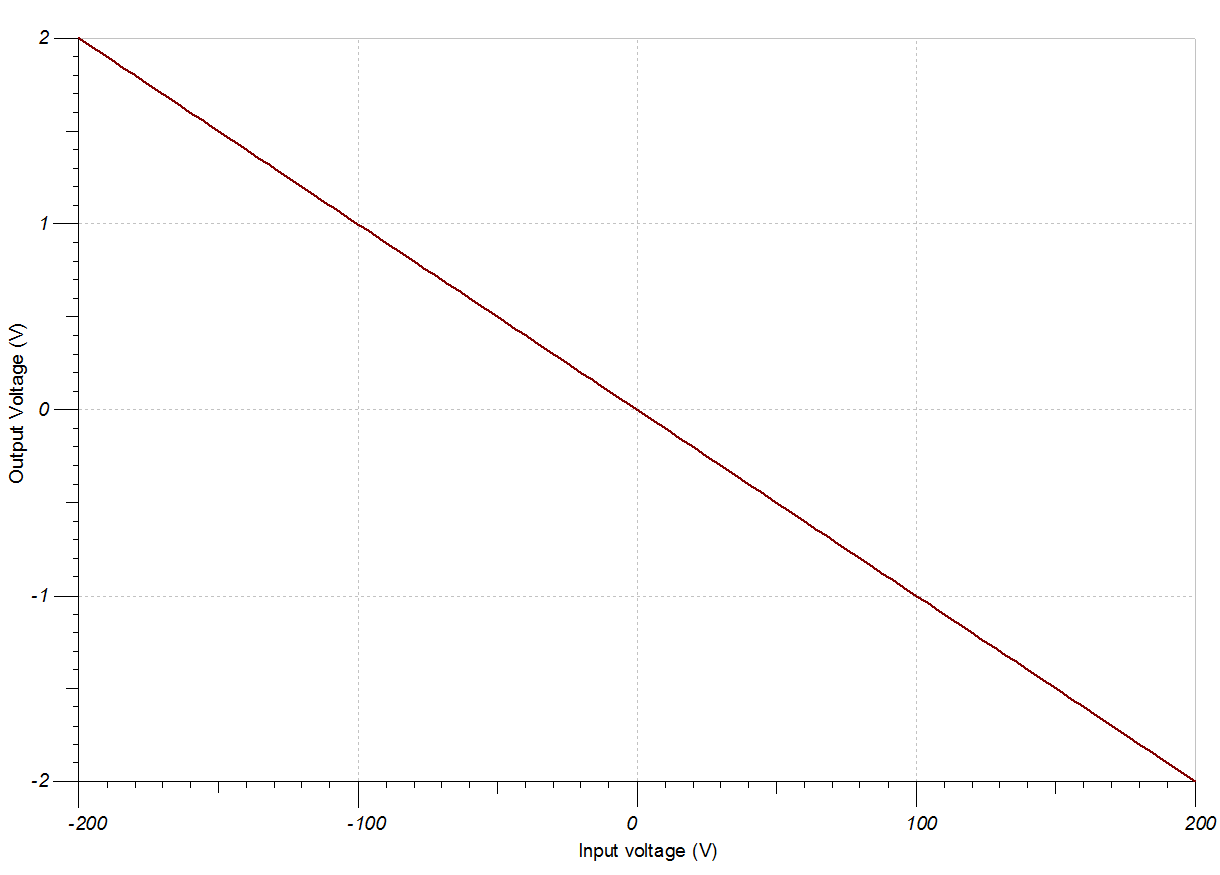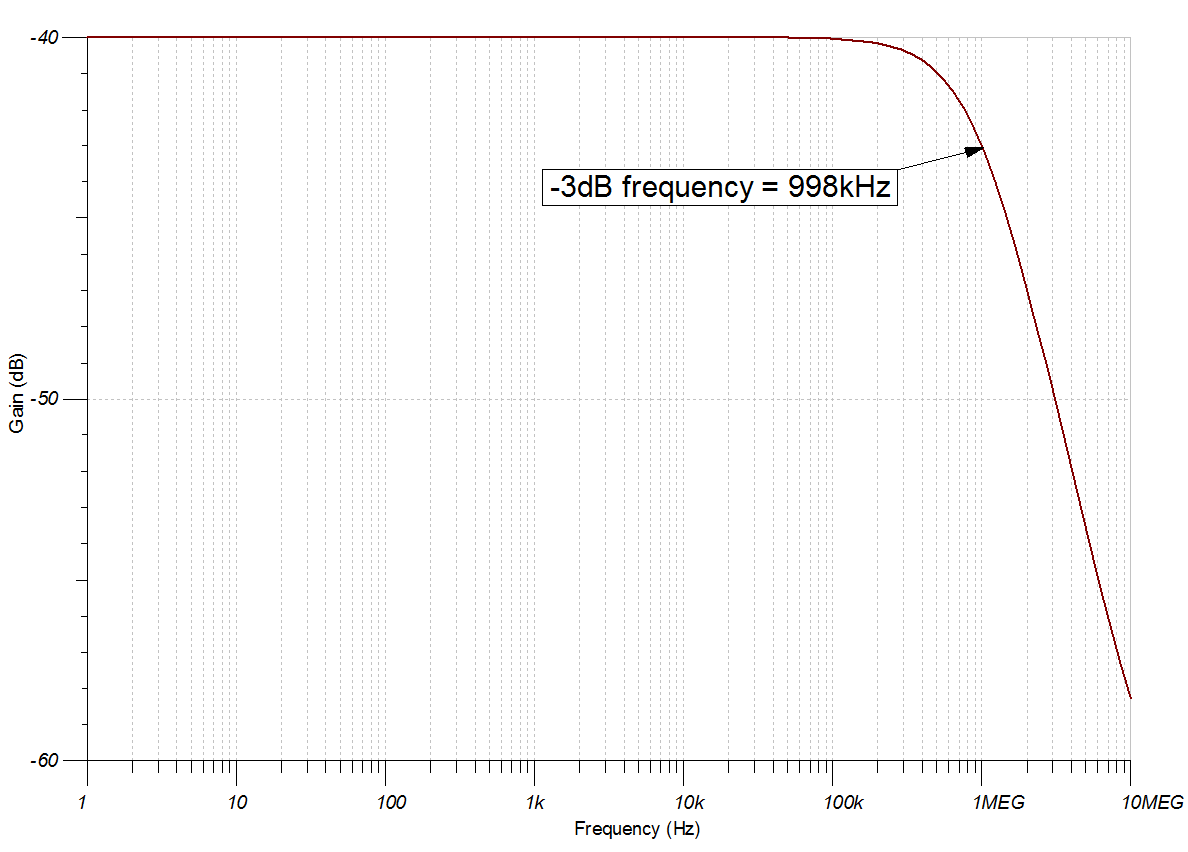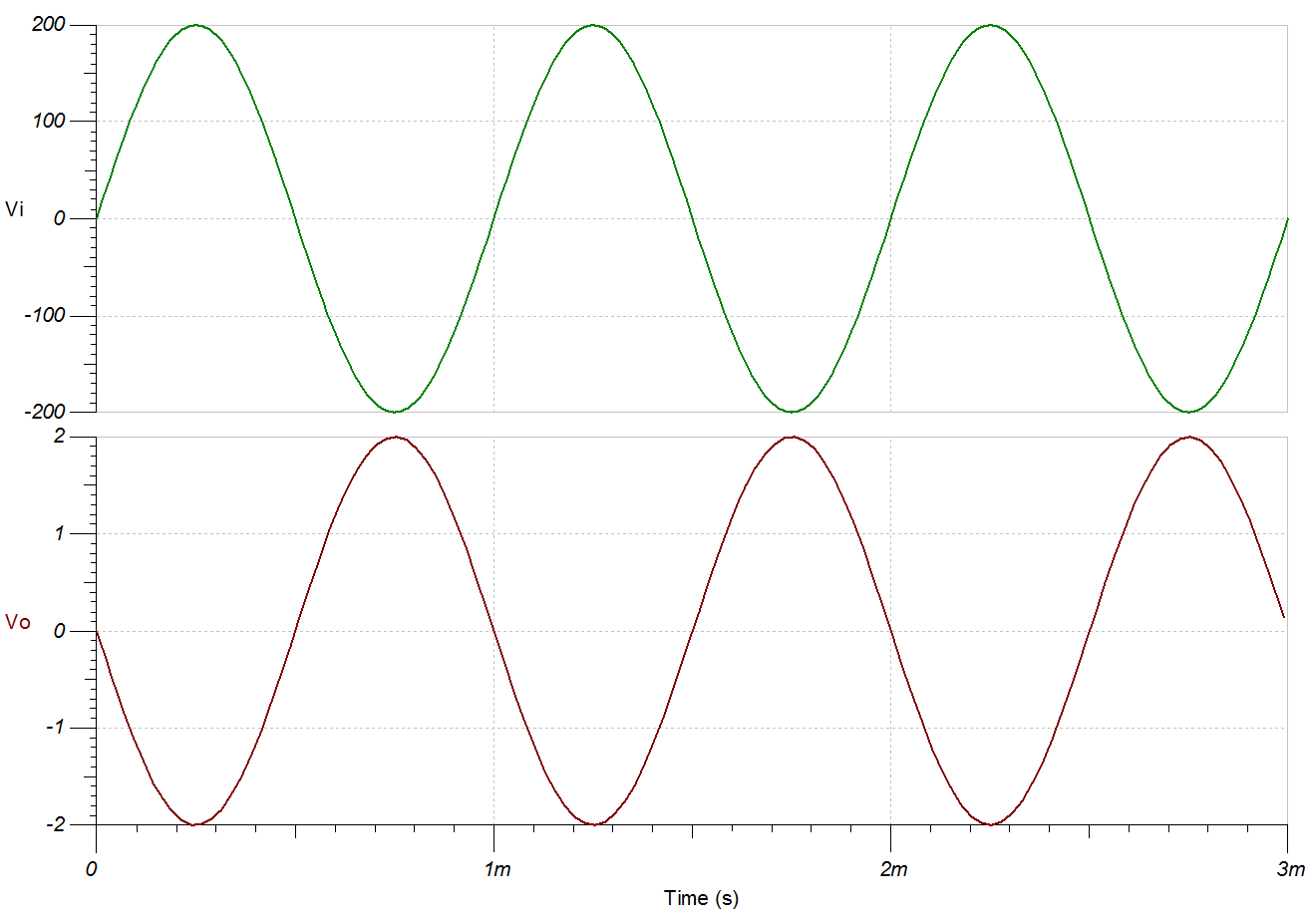SBOA283 March   2021

Design Goals

InputOutputBWGainSupply
ViMinViMaxVoMinVoMaxfpGVccVee
–200V200V–2V2V1MHz–40dB2.5V–2.5V

Design Description

This circuit inverts the input signal, Vi, and applies a signal gain of –40dB. The common-mode voltage of an inverting amplifier is equal to the voltage applied to the non-inverting input, which is ground in this design.Design Notes

1. The common-mode voltage in this circuit does not vary with input voltage.
2. The input impedance is determined by the input resistor. Make sure this value is large when compared to the output impedance of the source.
3. Using high-value resistors can degrade the phase margin of the circuit and introduce additional noise in the circuit. The capacitor in parallel with R2 provides filtering and improves stability of the circuit if high-value resistors are used for both the input and feedback resistances.
4. Avoid placing capacitive loads directly on the output of the amplifier to minimize stability issues.
5. Small-signal bandwidth is determined by the noise gain (or non-inverting gain) and op amp gain-bandwidth product (GBP).
6. Large signal performance may be limited by slew rate. Therefore, check the maximum output swing versus frequency plot in the data sheet to minimize slew-induced distortion.
7. For more information on op amp linear operating region, stability, slew-induced distortion, capacitive load drive, driving ADCs, and bandwidth see the Design References section.
8. Note that higher input voltage levels may require the use of multiple resistors in series to help reduce the voltage drop across the individual resistors. For more information, see the Design References section.

Design Steps

The transfer function of this circuit follows:

Equation 1. ${V}_{o}={V}_{i}×\left(–\frac{{R}_{2}}{{R}_{1}}\right)$
1. Calculate the gain required for the circuit.
Equation 1.
2. Choose the starting value of R1.
Equation 1. ${R}_{1}=100kΩ$
3. Calculate for a desired signal attenuation of 0.01 V/V.
Equation 1.
4. Select the feedback capacitor, C1, to meet the circuit bandwidth.
Equation 1. ${\text{C}}_{\text{1}}\text{≤}\frac{\text{1}}{{\text{2π × R}}_{\text{2}}{\text{× f}}_{\text{p}}}\text{→}$C112π × 1kΩ × 1MHz ≤ 159.15pF ≈ 160pF (Standard Value)
5. Calculate the minimum slew rate required to minimize slew-induced distortion.
Equation 1. SR > 2π× 1MHz × 2V= 12.6VμS
• SRLMV861 = 18V/µs; therefore, it meets this requirement.
6. Calculate the circuit bandwidth to ensure it meets the 1-MHz requirement. Be sure to use the noise gain, NG, or non-inverting gain, of the circuit.
Equation 1. BW =  GBP NG  =  30MHz 1.01 V V = 29.7MHz
• BWLMV861 = 30MHz; therefore, it meets this requirement.
7. If C1 is not used to limit the circuit bandwidth, to avoid stability issues ensure that the zero created by the gain setting resistors and input capacitance of the device is greater than the bandwidth of the circuit.
Equation 1.
• Ccm and Cdiff are the common-mode and differential input capacitance of the LMV861, respectively.

Design Simulations

DC Simulation ResultsAC Simulation ResultsTransient Simulation Results

A 1-kHz, 400-Vpp input sine wave yields a 4-Vpp output sine wave.Design References

1. See Analog Engineer's Circuit Cookbooks for the comprehensive TI circuit library.
2. SPICE Simulation File SBOC522.
3. TI Precision Labs
4. For more information on circuits with larger input voltages, see Considerations for High-Voltage Measurements.

Design Featured Op Amp

LMV861
Vss2.7V to 5.5V
VinCM(Vee – 0.1V) to (Vcc – 1.1V)
VoutRail-to-rail
Vos0.273mV
Iq2.25mA
Ib0.1pA
UGBW30MHz
SR18V/µs
#Channels1, 2
www.ti.com/product/LMV861

Design Alternate Op Amp

TLV9002OPA377
Vss1.8V to 5.5V2.2V to 5.5V
VinCMRail-to-railRail-to-rail
VoutRail-to-railRail-to-rail
Vos0.4mV0.25mV
Iq0.06mA0.76mA
Ib5pA0.2pA
UGBW1MHz5.5MHz
SR2V/µs2V/µs
#Channels1, 2, 41, 2, 4
www.ti.com/product/TLV9002www.ti.com/product/OPA377